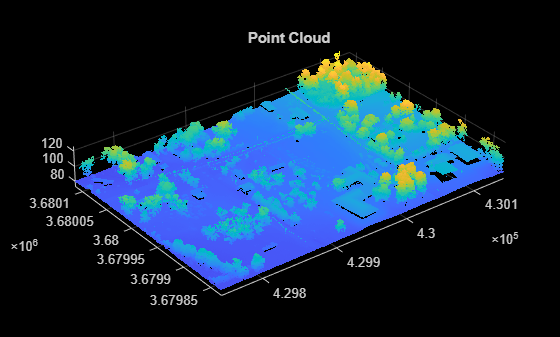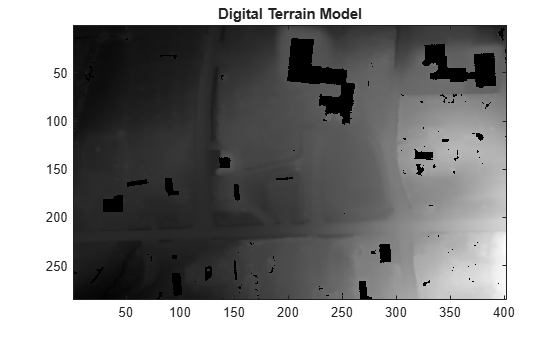# pc2dem

Create digital elevation model (DEM) of point cloud data

## Syntax

``elevModel = pc2dem(ptCloudIn)``
``elevModel = pc2dem(ptCloudIn,gridResolution)``
``[elevModel,xlimits,ylimits] = pc2dem(___)``
``[___] = pc2dem(___,Name,Value)``

## Description

example

````elevModel = pc2dem(ptCloudIn)` creates and returns a digital elevation model (DEM) `elevModel` for the input point cloud. The output matrix contains generalized elevation information of the input point cloud. For more information, see Algorithms.```
````elevModel = pc2dem(ptCloudIn,gridResolution)` additionally specifies the dimensions of the grid element.```
````[elevModel,xlimits,ylimits] = pc2dem(___)` additionally returns the x- and y-limits of the DEM, using any combination of input arguments from previous syntaxes.```

example

````[___] = pc2dem(___,Name,Value)` specifies options using one or more name-value arguments. For example, `'CornerFillMethod','min'` specifies for the function to compute the generalized elevation values for the grid corners in the DEM as the minimum elevation in the default search radius of each grid corner.```

## Examples

collapse all

Create a `lasFileReader` object to read point cloud data stored in `aerialLidarData.laz` file.

```fileName = fullfile(toolboxdir("lidar"),"lidardata","las", ... "aerialLidarData.laz"); lasReader = lasFileReader(fileName);```

Read point cloud data from the file using the `readPointCloud` function.

`ptCloud = readPointCloud(lasReader);`

Visualize the point cloud data.

```figure pcshow(ptCloud.Location) title("Point Cloud")```Segment the ground points from the point cloud data.

`groundPtsIdx = segmentGroundSMRF(ptCloud);`

Extract the ground points.

`ptCloudWithGround = select(ptCloud,groundPtsIdx);`

Visualize the ground points.

```figure pcshow(ptCloudWithGround.Location) title("Ground Points")```Create a digital terrain model (DTM) from the segmented ground points.

`terrainModel = pc2dem(ptCloudWithGround);`

Visualize the DTM.

```figure imagesc(terrainModel) colormap(gray) title("Digital Terrain Model")```Create a `lasFileReader` object to read aerial point cloud data from "`aerialLidarData.laz"`.

```fileName = fullfile(toolboxdir("lidar"),"lidardata","las", ... "aerialLidarData.laz"); lasReader = lasFileReader(fileName);```

Read the point cloud data of the first return of the lidar sensor from the LAS file using the `readPointCloud` function.

`ptCloud = readPointCloud(lasReader,"LaserReturn",1);`

Create a digital surface model (DSM) of the point cloud with a grid element resolution of 1.1 meters.

```gridRes = 1.1; surfaceModel = pc2dem(ptCloud,gridRes,"CornerFillMethod","max");```

Define the location of the illumination source.

```azimuthAng = 135; zenithAng = 45;```

Compute the directional gradients of the DSM using the `imgradientxy` function.

`[gx,gy] = imgradientxy(surfaceModel,"sobel");`

Normalize the gradients using the grid element resolution.

```gx = gx/(8*gridRes); gy = gy/(8*gridRes);```

Compute the slope and aspect of the DSM.

```slopeAngle = atand(sqrt(gx.^2 + gy.^2)); aspectAngle = atan2d(gy,-gx); aspectAngle(aspectAngle < 0) = aspectAngle(aspectAngle < 0) + 360;```

Calculate the hillshade using the algorithm from Esri®. A hillshade is a 3-D grayscale representation of a surface, with the relative position of the illumination source taken into account when shading the image.

```h = 255.0*((cosd(zenithAng).*cosd(slopeAngle)) ... + (sind(zenithAng).*sind(slopeAngle).*cosd(azimuthAng - aspectAngle))); h(h < 0) = 0;```

Visualize the hillshade of the DSM.

```figure imagesc(h) colormap(gray)```## Input Arguments

collapse all

Input point cloud, specified as a `pointCloud` object.

Resolution of the grid element along the xy-axes, specified as a two-element vector of the form [x y], or as a scalar for square elements. Values for this argument must be positive real numbers.

Data Types: `single` | `double` | `int8` | `int16` | `int32` | `int64` | `uint8` | `uint16` | `uint32` | `uint64`

### Name-Value Arguments

Specify optional pairs of arguments as `Name1=Value1,...,NameN=ValueN`, where `Name` is the argument name and `Value` is the corresponding value. Name-value arguments must appear after other arguments, but the order of the pairs does not matter.

Before R2021a, use commas to separate each name and value, and enclose `Name` in quotes.

Example: `'CornerFillMethod','min'` selects the type of method to compute the generalized elevation values for each grid corner in the DEM.

Method for grid corner the generalized elevation value calculation, specified as a character vector or a string scalar. The list of supported methods and how they must be specified is as follows:

• `'min'` — Minimum elevation of all the points in the search radius

• `'max'` — Maximum elevation of all the points in the search radius

• `'mean'` — Mean elevation of all the points in the search radius

• `'idw'` — Inverse distance weighted (IDW) mean elevation of all the points in the search radius

Data Types: `char` | `string`

Radius of search region around each grid corner, specified as a positive scalar.

Data Types: `single` | `double` | `int8` | `int16` | `int32` | `int64` | `uint8` | `uint16` | `uint32` | `uint64`

Filter size for IDW interpolation to fill unfilled grid corners, specified as a positive odd integer.

Data Types: `single` | `double` | `int8` | `int16` | `int32` | `int64` | `uint8` | `uint16` | `uint32` | `uint64`

## Output Arguments

collapse all

Digital elevation model, returned as an M-by-N matrix of real values. The values of M and N are computed based on point cloud limits along the xy-axes and the `gridResolution`.

x-axis limits of the elevation model, returned as a two-element real-valued vector.

y-axis limits of the elevation model, returned as a two-element real-valued vector.

## Algorithms

The function uses a local binning algorithm to create a digital elevation model (DEM) of the point cloud data. The algorithm assumes that the elevation of points is along the z-axis.

Local Binning Algorithm:

• Divide the point cloud into a grid along the xy-dimensions (bird's eye view). Specify the grid dimensions using the `gridResolution` argument.

• Utilize the elevation information of all points within a circular region around each grid corner to compute generalized grid values. You can specify the search radius and computation method using the `'SearchRadius'` and `'CornerFillMethod'` name-value arguments, respectively.

• If there are no points within the circular region, the algorithm does not compute a value and those grid corners remain unfilled. The function represents them as `NaN`. The algorithm uses inverse distance weighted (IDW) interpolation to fill the unfilled grid corners. To specify the filter size for the IDW interpolation method, use the `'FilterSize'` name-value argument.

## Version History

Introduced in R2021b